Investigating Euler's Polyhedral Formula Using Original Sources - Euler's Polyhedral Formula

Author(s):

Proposition 4 is the most famous one; we have arrived at "Euler's Polyhedral Formula".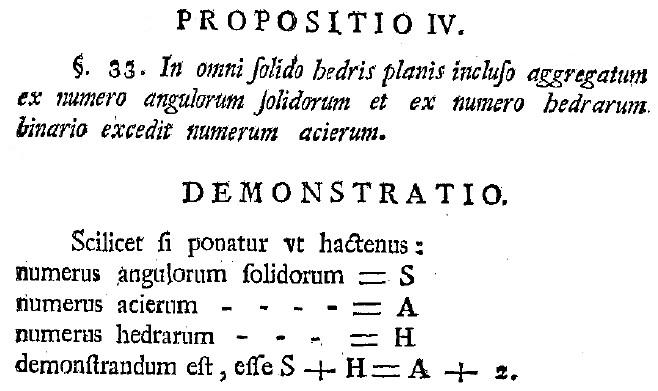Figure 9: Euler's Polyhedral Formula

After reminding us what the variables stand for (a good mathematical practice in general!), Euler writes the result

S + H = A + 2,

which we would write in modern notation as

V + F = E + 2.

Euler admits right away that he does not have a firm proof of this theorem, but in an effort to convince the reader, he works through eight classes of examples. He refers to figures at the back of the article, but in reality each picture is just meant as an example of the class for which Euler writes a proof. In the first four classes of examples, Euler proves that this formula holds for pyramids, wedges, prisms, and cupola-like objects.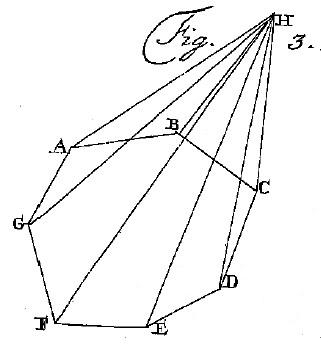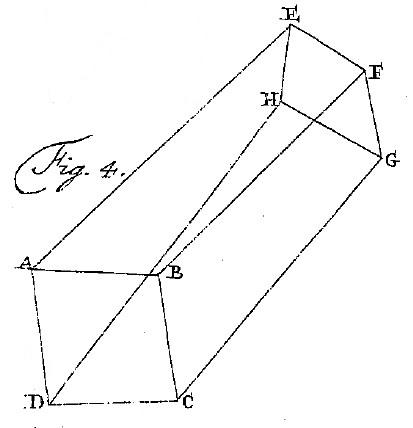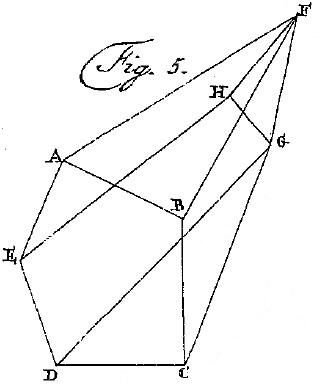Figure 10: Pyramids, Wedges, Prisms, and Cupola-like Solids

These first four classes actually form an elegant progression of solids of increasingly complexity:

• Pyramids have a base that is an n-gon, all of whose vertices are connected via edges to a single point;
• Wedges have an n-gon base with vertices connected to one of the two endpoints of a line segment;
• Prisms have an n-gon base with vertices connected to the vertices of another n-gon;
• Cupola-like solids have an n-gon base with vertices connected to the vertices of an m-gon, where m is any positive integer except 1, 2, or n (as these cases were previously covered).

Euler concludes his demonstration by verifying the formula holds for the Platonic solids.

Working through these verifications for specific cases (such as finding the values of V, E, and F, for the pentagonal prism and checking that the formula holds) and for general cases (such as for an n-gonal prisms, finding values for V, E, and F in terms of n, and checking that the formula holds) is invaluable practice for students.

There is also an important discussion to be had, centered on a pair of questions:

1. Are you convinced that Euler's formula is true?
2. Did Euler prove that the polyhedral formula is true?

After gathering answers to these two questions, students can explore how the answers to these two questions are related. For example, ask students: If you are convinced, is it a proof? What should be the role of a proof, besides convincing the reader? What if the proof is too complicated and does not convey understanding -- is a series of examples better? Can a series of examples be converted into a proof? There is a wealth of good discussion material to be had here.

Also interesting are the three corollaries to Proposition 4. They are: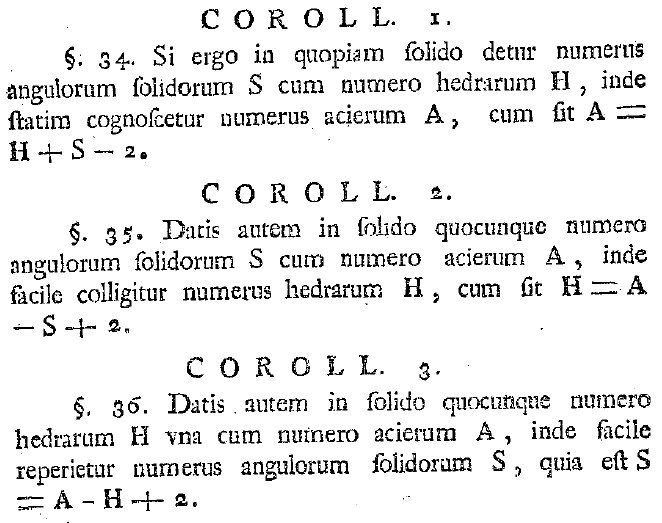Figure 11: Polyhedral Formula Corollaries

In short, using modern terminology:

Corollary 1: E = F + V - 2
Corollary 2: F = E - V + 2
Corollary 3: V = E - F + 2

What is particularly striking about the four different formulations of the polyhedral formula is that among them, we do not find the formula written in the form that it is typically found today:

V - E + F = 2

Reading "between the lines", we hypothesize that this is due to a shift in the way we interpret the significance of these equations. To Euler, perhaps, these equations provide a method to solve for any one of these three variables if given the values of the other two. However, a more modern interpretation of the significance of this equation is that the quantity V - E + F is the same (it is equal to 2) for any solid in the classes that Euler considered; the number 2 is a characteristic (or an invariant) for these classes of solids. Other solids (such as tori) will result in a different characteristic number at the end of such a formula.